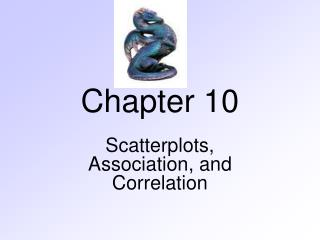Download PresentationChapter 10Chapter 10 - PowerPoint PPT Presentation

Download PresentationChapter 10
An Image/Link below is provided (as is) to download presentation

Download Policy: Content on the Website is provided to you AS IS for your information and personal use and may not be sold / licensed / shared on other websites without getting consent from its author. While downloading, if for some reason you are not able to download a presentation, the publisher may have deleted the file from their server.

- - - - - - - - - - - - - - - - - - - - - - - - - - - E N D - - - - - - - - - - - - - - - - - - - - - - - - - - -
Presentation Transcript

1. Chapter 10 Scatterplots, Association, and Correlation

2. Scatterplots • What we look for: • Direction • Form • Strength • Outliers

3. Scatterplots - Direction • Negative - a pattern that runs upper left to lower right. • Positive – a pattern that runs lower left to upper right.

4. Scatterplots - Form • Linear – the pattern follows a straight line. • Non-linear – the pattern does not follow a straight line.

5. Scatterplots - Strength • Strong association – the data points are “close” together. • Weak association – the data points are spread apart.

6. Scatterplots - Outliers • As before we need to note outliers and investigate if they are a point that we need to remove from the data set.

7. Scatterplots

8. Variable Roles • Put explanatory variable on x-axis. • Hope this variable will explain or predict. • Put response variable on y-axis. • We think this variable will show a response. • Its our choice as to which variable we think will play each role.

9. Variable Roles

10. Correlation • A numerical measure of the direction and strength of a linear association. • Like standard deviation was a numerical measure of spread.

11. Correlation Coefficient - Facts • The correlation coefficient is denoted by the letter r. • Safe to assume r is always correlation in this class. • The sign of the correlation coefficient give the direction of the association. • Positive is positive and negative is negative.

12. Correlation Coefficient - Facts • The correlation coefficient is always between -1 and +1. • A low correlation is closer to zero and strong closer to either -1 or +1. • Ex. r = 0.21 or -0.21 (weak), r = -0.98 or 0.98(strong). • If correlation is equal to exactly -1 or +1 then the data points all fall on an exact straight line.

13. Correlation Coefficient - Facts • Correlation coefficient has no units. • The correlation is just that the correlation. • Learn it on its own scale, not as a percentage. • Correlation doesn’t change if center or scale of original data is changed. • Depends only on the z-score.

14. What is STRONG/WEAK? • Again a judgment call. • Rule of thumb: • 0 to +/- 0.5 Weak • +/- 0.5 to +/- 0.80 Moderate • +/- 0.8 to +/- 1.0 Strong

15. Computing Correlation • Use your technology to help you find this number. • Calculator

16. Price of Homes Based on Size (in Square Feet)

17. Models for Data • Draw a line to summarize the relationship between two variables • This line is called the regression line. • Explanatory variable (x) • Response variable (y)

18. Correlation and the Line Price of Homes Based on Square Feet Price = -75.47 + 0.69SQFT R2 = 80.2%

19. Regression line • Explains how the response variable (y) changes in relation to the explanatory variable (x) • Use the line to predict value of y for a given value of x

20. Regression line equation

21. Regression line equation • a = slope of line. For every unit increase in x, y changes by the amount of the slope. • b = y-intercept of line. The value of y when x = 0.

22. Prediction • Use the regression equation to predict y from x. • Ex. What is the predicted calorie count when the serving size is = 150 grams? • Ex. What is the predicted calorie count when the serving size is = 300 grams?

23. Properties of regression line • r is related to the value of b1 • r has the same sign as b1 • One standard deviation change in x corresponds to r times one standard deviation change in y • The regression line always goes through the point

24. Properties of regression line • r2 • Percent of variation in y that is explained by the least squares regression of y on x • The higher the value of r2, the more the regression line explains the changes that occur in the y variable • The higher the values of r2, the better the regression line fits the data • 0  r2  1 since -1  r 1

25. Cautions about regression • Linear relationship only • Not resistant • Extrapolation • Predicting y when x value is outside the original data